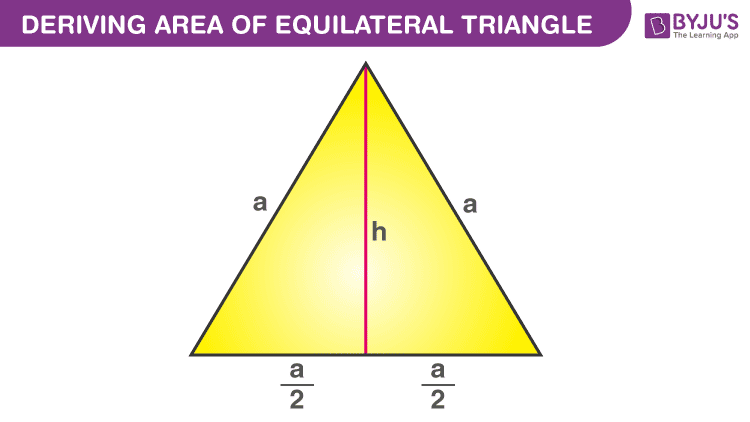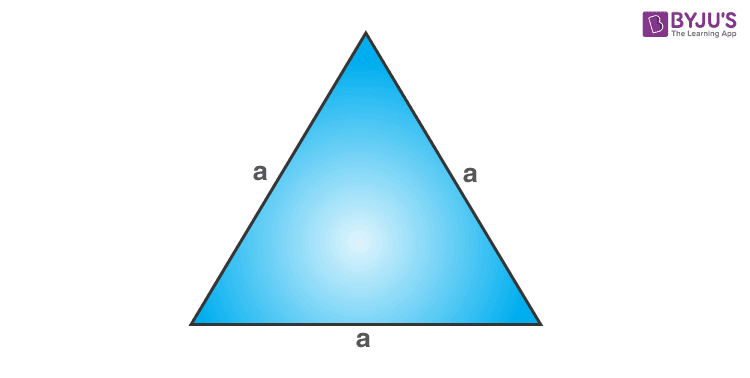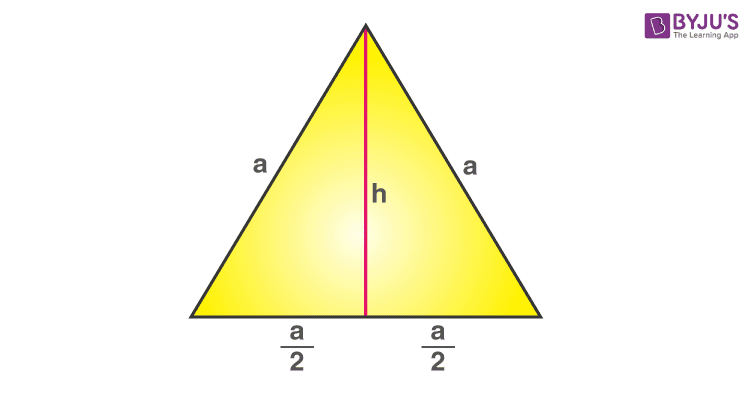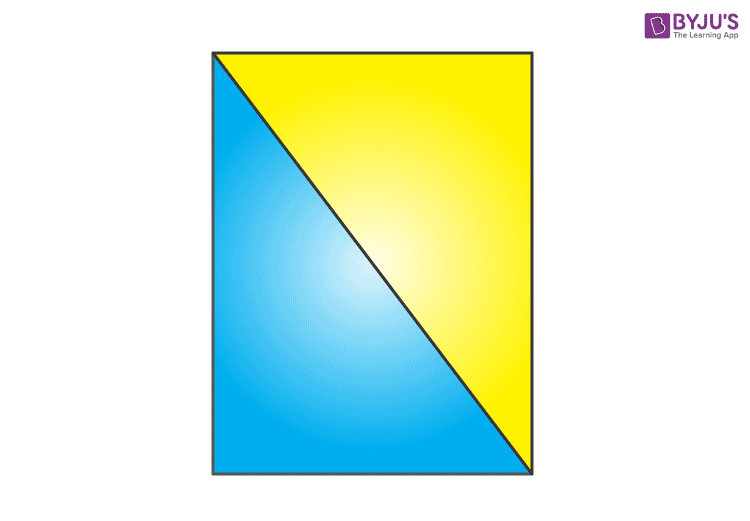# Area Of Equilateral Triangle

The area of an equilateral triangle is the amount of space that it occupies in a 2-dimensional surface. To recall, an equilateral triangle is a triangle whose all sides are equal and the measure of all the internal angles is 60°. So, an equilateral triangle’s area can be calculated if the length of its side is known.

## Area of an Equilateral Triangle Formula

The formula for the area of an equilateral triangle is given as:

 Area of Equilateral Triangle (A) = (√3/4)a2

Where a = length of sides

## Derivation for Area of Equilateral Triangle

There are three methods to derive the formula for the area of equilateral triangles which are:

• Using basic triangle formula
• Using rectangle construction
• Using trigonometry

### Deriving Area of Equilateral Triangle Using Basic Triangle Formula

Take an equilateral triangle of the side “a” units. Then draw a perpendicular bisector to the base of height “h”.Deriving Area Of Equilateral Triangle

Now,

Area of Triangle = ½ × base × height

Here, base = a, and height = h

Now, apply Pythagoras Theorem in the triangle.

a2 = h2 + (a/2)2

⇒ h2=a2–a2/4

⇒ h2=(3a2)/4

Or, h=½(√3a)

Now, put the value of “h” in the area of the triangle equation.

So,

Area of Triangle = ½ × base × height

⇒ A = ½ × a × ½(√3a)

Or, Area of Equilateral Triangle = ¼(√3a2)

### Deriving Area of Equilateral Triangle Using Rectangle Construction

Consider an equilateral triangle having sides equal to “a”.Equilateral Triangle

• Now, drop a straight line from the top triangle vertex to the midpoint of the base of the triangle, thus, dividing the base into two equal halves.Area Of Equilateral Triangle

• Now cut along the straight line and move the other half of the triangle to form the rectangle.How to find Area of Equilateral Triangle?

Here, the length of the equilateral triangle is considered to be ‘a’ and the height as ‘h’

So the area of an equilateral triangle = Area of a rectangle = ½×a×h …………. (i)

Half of the rectangle is a right-angled triangle as it can be seen from the figure above.

Thereby, applying the Pythagoras Theorem

⇒ a2=h2+ (a/2)2

⇒ h2=(3/4)a2

⇒ h = (√3/2)a …………..(ii)

Substituting the value of (ii) in (i), we have:

Area of an Equilateral Triangle

=(½)×a×(√3/2)a

=(√3/4)a2

### Deriving Area of Equilateral Triangle Using Trigonometry

If two sides of a triangle are given, then the height can be calculated using trigonometric functions. Now, the height of a triangle ABC will be-

h = b•Sin C = c•Sin A = a•Sin B

Now, area of ABC = ½ × a × (b • sin C) = ½ × b × (c • sin A) = ½ × c (a • sin B)

Now, since it is a equilateral triangle, a = b = c = 60°

So,

Area = ½ × a × (a • Sin 60°) = ½ × a2 × Sin 60° = ½ × a2 × √3/2

So, Area of Equilateral Triangle = (√3/4)a2

Below is a brief recall about equilateral triangles:

### What is an Equilateral Triangle?

There are mainly three types of triangles which are scalene triangles, equilateral triangles, and isosceles triangles. An equilateral triangle has all the three sides equal and all angles equal to 60°. All the angles in an equilateral triangle are congruent.

### Properties of Equilateral Triangle

An equilateral triangle is the one in which all three sides are equal. It is a special case of the isosceles triangle where the third side is also equal. In the triangle ABC, AB = BC = CA.

Some important properties of an equilateral triangle are:

• An equilateral triangle is a triangle in which all three sides are equal.
• Equilateral triangles are also equiangular, which means, all three internal angles are also equal to each other and the only value possible is 60° each.
• It is a regular polygon with 3 sides.
• A triangle is equilateral if and only if the circumcenters of any three of the smaller triangles have the same distance from the centroid.
• A triangle is equilateral if and only if any three of the smaller triangles have either the same perimeter or the same inradius.
• The area of an equilateral triangle is basically the amount of space occupied by an equilateral triangle.
• In an equilateral triangle, the median, angle bisector and perpendicular are all the same and can be simply termed as the perpendicular bisector due to congruence conditions.
• The ortho-centre and centroid of the triangle is the same point.
• In an equilateral triangle, median, angle bisector, and altitude for all sides are all the same and are the lines of symmetry of the equilateral triangle.
• The area of an equilateral triangle is √3 a2/ 4
• The perimeter of an equilateral triangle is 3a.

### Example Questions Using the Equilateral Triangle Area Formula

Question 1: Find the area of an equilateral triangle whose perimeter is 12 cm?

Solution:

Given: Perimeter of an equilateral triangle = 12 cm

As per formula: Perimeter of the equilateral triangle = 3a, Where a is the side of the equilateral triangle.

Step 1: Find the side of an equilateral triangle using perimeter.

3a = 12

a = 4

The side is 4 cm.

Step 2: Find the area of an equilateral triangle using formula.

Area, A = √3 a2/ 4 sq units

= √3 (4)2/ 4

= 4√3

Therefore, the area of given equilateral triangle is 4√3 cm2

Question 2: What is the area of an equilateral triangle whose side is 8cm?

Solution:

The area of the equilateral triangle = √3 a2/ 4 cm2

= √3 × (82)/ 4 cm2

= √3 × 16 cm2

= 16 √3 cm2

Question 3: Find the area of an equilateral triangle whose side is 7 cm.

Solution:

Given,

Side of the equilateral triangle = a = 7 cm

Area of an equilateral triangle = √3 a2/ 4

= √3/4 × 72 cm2

= √3/4 × 49 cm2

= 21.21762 cm2

Question 4: Find the area of an equilateral triangle whose side is 28 cm.

Solution:

Given,

Side of the equilateral triangle (a) = 28 cm

We know,

Area of an equilateral triangle = √3 a2/ 4

= √3/4 × 282 cm2

= √3/4 × 784 cm2

= 339.48196 cm2

### What is an Equilateral Triangle?

An equilateral triangle can be defined as a special type of triangle whose all the sides and internal angles are equal. In an equilateral triangle, the measure of internal angles is 60 degrees.

### What does the Area of an Equilateral Triangle Mean?

The area of an equilateral triangle is defined as the amount of space occupied by the equilateral triangle in the two-dimensional area.

### What is the Formula for Area of Equilateral Triangle?

To calculate the area of an equilateral triangle, the following formula is used:

A = ¼(√3a2)

### What is the Formula for Perimeter of Equilateral Triangle?

The formula to calculate the perimeter of a equilateral triangle is:

P = 3a

#### 1 Comment

1. S KHERIWALA

WELL EXPLAINED